0

# XGBoost和LightGBM时间序列预测对比

XGBoost和LightGBM都是目前非常流行的基于决策树的机器学习模型，它们都有着高效的性能表现，但是在某些情况下，它们也有着不同的特点。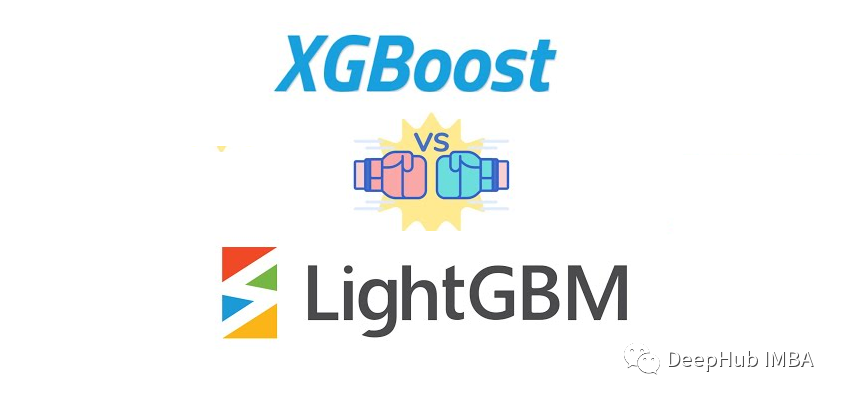## XGBoost和LightGBM简单对比

LightGBM相较于xgboost在训练速度方面有明显的优势。这是因为LightGBM使用了一些高效的算法和数据结构，比如直方图算法和基于梯度单边采样算法（GOSS），这些算法使得LightGBM在训练大规模数据集时速度更快。

xgboost在处理一些不规则数据时更加鲁棒，比如一些缺失值和异常值。而LightGBM在这方面相对较弱。

xgboost的参数比较多，需要根据实际情况进行调整。而LightGBM的参数相对较少，大多数情况下使用默认参数即可。

## XGBoost和 LightGBM 算法对比

XGBoost和 LightGBM 都是基于决策树的梯度提升框架，它们的核心思想都是通过组合多个弱学习器来提升模型的预测能力。它们在实现上有很多相似之处，但在算法方面有一些明显的不同：

xgboost 将数据按特征进行划分，然后将每个特征分配到不同的节点上进行计算。这种方法可以有效提高训练速度，但需要额外的通信和同步开销。

LightGBM 将数据按进行划分，然后将每个分块分配到不同的节点上进行计算。这种方法避免了通信和同步开销，但需要额外的内存空间。

xgboost 会自动将缺失值分配到左右子树中概率更高的那一边。这种方法可能会引入一些偏差，但对于处理缺失值较多的数据集比较有效。

LightGBM 则采用的方法称为 Zero As Missing（ZAM），它将所有的缺失值都视为一个特殊的取值，并将其归入其中一个子节点中。这种方法可以避免偏差，但需要更多的内存空间。

LightGBM 在训练速度方面具有显著优势，这是因为它使用了 GOSS 和直方图算法，减少了计算量和内存消耗。而 xgboost 的计算速度相对较慢，但是在处理较小的数据集时表现良好。

## 电力能源消耗预测

1、预处理

`````` import pandas as pd
import matplotlib.pyplot as plt

print(df.isna().sum())
``````

“LCLid”是标识每个家庭的唯一字符串，“Date”就是我们的时间索引，“KWH”是在该日期花费的总千瓦时数，没有任何缺失值。由于我们想要以一般方式而不是以家庭为单位来预测耗电量，所以我们需要将结果按日期分组并平均千瓦时。

`````` df_avg_consumption = df.groupby("Date")["KWH"].mean()
df_avg_consumption = pd.DataFrame({"date": df_avg_consumption.index.tolist(), "consumption": df_avg_consumption.values.tolist()})
df_avg_consumption["date"] = pd.to_datetime(df_avg_consumption["date"])
print(f"From: {df_avg_consumption['date'].min()}")
print(f"To: {df_avg_consumption['date'].max()}")
``````

`````` df_avg_consumption.plot(x="date", y="consumption")
``````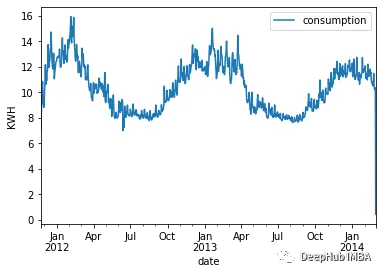`````` df_avg_consumption.query("date > '2012-01-01' & date < '2013-01-01'").plot(x="date", y="consumption")
``````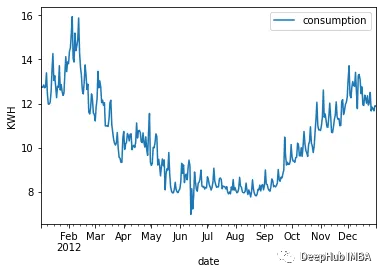`````` df_avg_consumption["day_of_week"] = df_avg_consumption["date"].dt.dayofweek
df_avg_consumption["day_of_year"] = df_avg_consumption["date"].dt.dayofyear
df_avg_consumption["month"] = df_avg_consumption["date"].dt.month
df_avg_consumption["quarter"] = df_avg_consumption["date"].dt.quarter
df_avg_consumption["year"] = df_avg_consumption["date"].dt.year

``````

' date '特征就变得多余了。但是在删除它之前，我们将使用它将数据集分割为训练集和测试集。与传统的训练相反，在时间序列中，我们不能只是以随机的方式分割集合，因为数据的顺序非常重要，所以对于测试集，将只使用最近6个月的数据。如果训练集更大，可以用去年全年的数据作为测试集。

`````` training_mask = df_avg_consumption["date"] < "2013-07-28"
print(training_data.shape)

print(testing_data.shape)
``````

`````` figure, ax = plt.subplots(figsize=(20, 5))
training_data.plot(ax=ax, label="Training", x="date", y="consumption")
testing_data.plot(ax=ax, label="Testing", x="date", y="consumption")
plt.show()
``````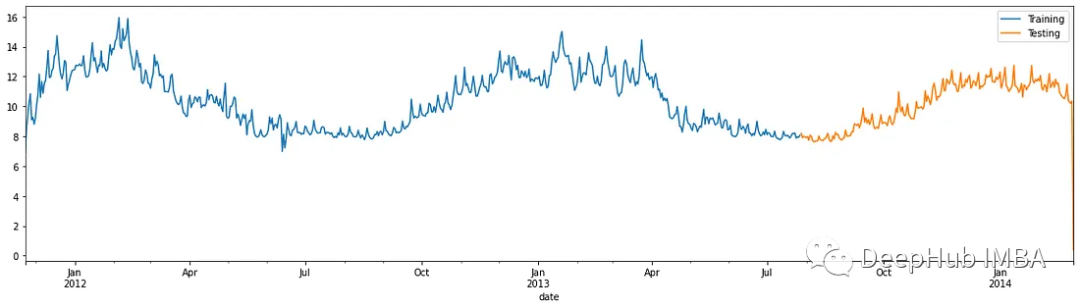`````` # Dropping unnecessary `date` column
training_data = training_data.drop(columns=["date"])
testing_dates = testing_data["date"]
testing_data = testing_data.drop(columns=["date"])

X_train = training_data[["day_of_week", "day_of_year", "month", "quarter", "year"]]
y_train = training_data["consumption"]

X_test = testing_data[["day_of_week", "day_of_year", "month", "quarter", "year"]]
y_test = testing_data["consumption"]
``````

2、训练模型

`````` from xgboost import XGBRegressor
import lightgbm as lgb
from sklearn.model_selection import TimeSeriesSplit, GridSearchCV

# XGBoost
cv_split = TimeSeriesSplit(n_splits=4, test_size=100)
model = XGBRegressor()
parameters = {
"max_depth": [3, 4, 6, 5, 10],
"learning_rate": [0.01, 0.05, 0.1, 0.2, 0.3],
"n_estimators": [100, 300, 500, 700, 900, 1000],
"colsample_bytree": [0.3, 0.5, 0.7]
}

grid_search = GridSearchCV(estimator=model, cv=cv_split, param_grid=parameters)
grid_search.fit(X_train, y_train)
``````

`````` # LGBM
cv_split = TimeSeriesSplit(n_splits=4, test_size=100)
model = lgb.LGBMRegressor()
parameters = {
"max_depth": [3, 4, 6, 5, 10],
"num_leaves": [10, 20, 30, 40, 100, 120],
"learning_rate": [0.01, 0.05, 0.1, 0.2, 0.3],
"n_estimators": [50, 100, 300, 500, 700, 900, 1000],
"colsample_bytree": [0.3, 0.5, 0.7, 1]
}

grid_search = GridSearchCV(estimator=model, cv=cv_split, param_grid=parameters)
grid_search.fit(X_train, y_train)
``````

3、评估

`````` from sklearn.metrics import mean_absolute_error, mean_absolute_percentage_error,\
mean_squared_error

def evaluate_model(y_test, prediction):
print(f"MAE: {mean_absolute_error(y_test, prediction)}")
print(f"MSE: {mean_squared_error(y_test, prediction)}")
print(f"MAPE: {mean_absolute_percentage_error(y_test, prediction)}")

def plot_predictions(testing_dates, y_test, prediction):
df_test = pd.DataFrame({"date": testing_dates, "actual": y_test, "prediction": prediction })
figure, ax = plt.subplots(figsize=(10, 5))
df_test.plot(ax=ax, label="Actual", x="date", y="actual")
df_test.plot(ax=ax, label="Prediction", x="date", y="prediction")
plt.legend(["Actual", "Prediction"])
plt.show()
``````

`````` # Evaluating GridSearch results
prediction = grid_search.predict(X_test)
plot_predictions(testing_dates, y_test, prediction)
evaluate_model(y_test, prediction)
``````

XGB：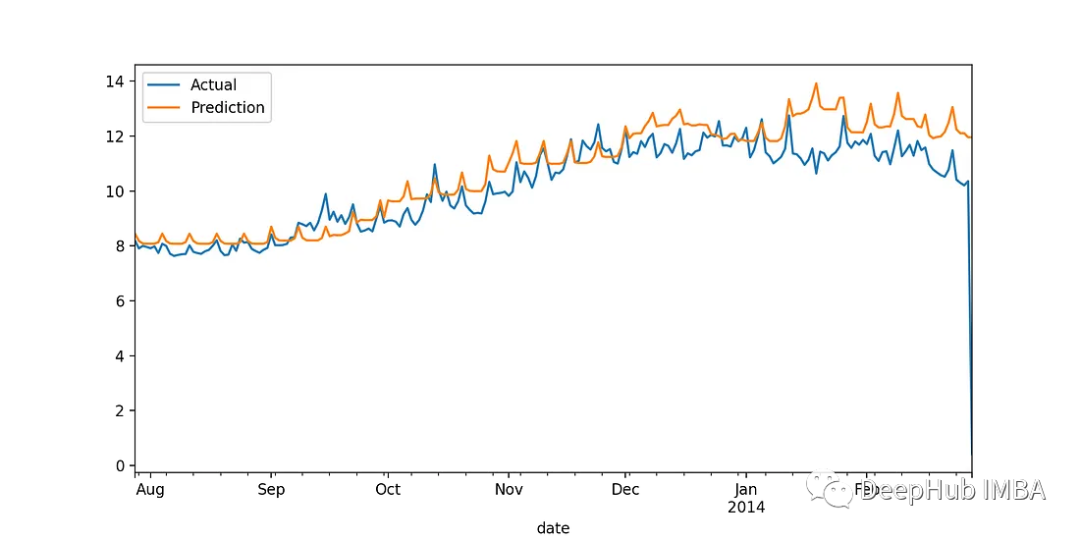LightGBM：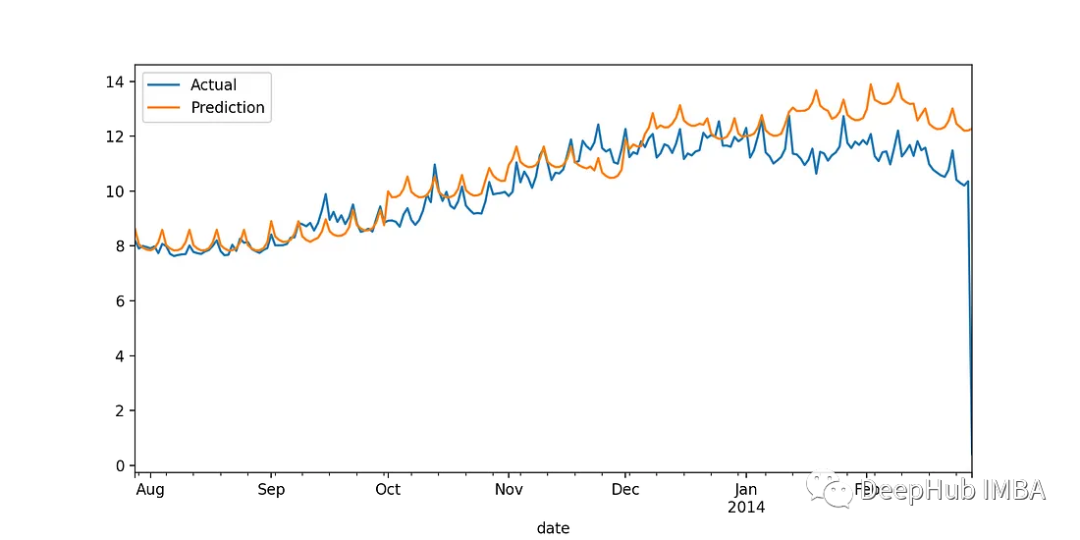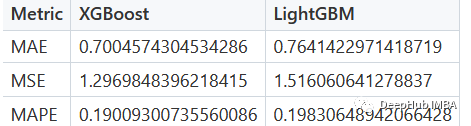## 使用外部辅助天气数据

`````` df_weather = pd.read_csv("london_weather.csv")
print(df_weather.isna().sum())
``````

`````` # Parsing dates
df_weather["date"] = pd.to_datetime(df_weather["date"], format="%Y%m%d")

# Filling missing values through interpolation
df_weather = df_weather.interpolate(method="ffill")

# Enhancing consumption dataset with weather information
df_avg_consumption = df_avg_consumption.merge(df_weather, how="inner", on="date")
``````

`````` # Dropping unnecessary `date` column
training_data = training_data.drop(columns=["date"])
testing_dates = testing_data["date"]
testing_data = testing_data.drop(columns=["date"])

X_train = training_data[["day_of_week", "day_of_year", "month", "quarter", "year",\
"mean_temp", "min_temp", "precipitation", "pressure",\
"snow_depth"]]
y_train = training_data["consumption"]

X_test = testing_data[["day_of_week", "day_of_year", "month", "quarter", "year",\
"mean_temp", "min_temp", "precipitation", "pressure",\
"snow_depth"]]
y_test = testing_data["consumption"]
``````

XGBoostLightGBM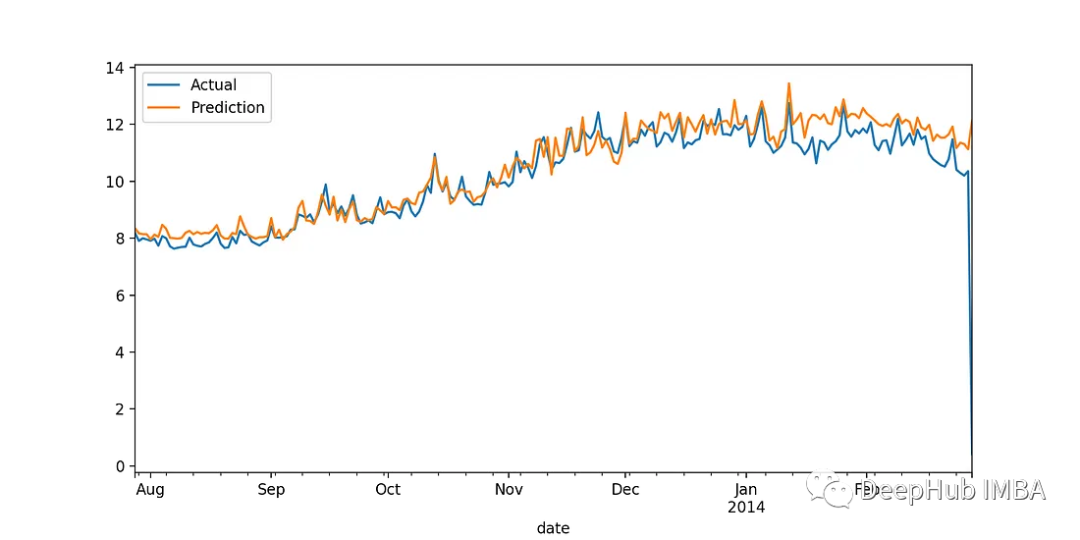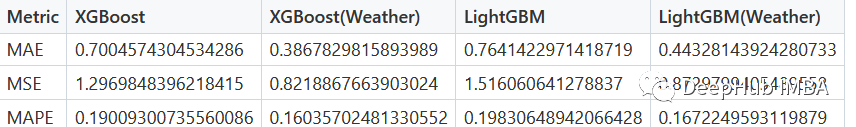## 总结

xgboost 和 LightGBM 都是优秀的梯度提升框架，它们各自具有一些独特的优点和缺点，选择哪一种算法应该根据实际应用场景和数据集的特征来决定。如果数据集中缺失值较多，可以选择 xgboost。如果需要处理大规模数据集并追求更快的训练速度，可以选择 LightGBM。如果需要解释模型的特征重要性，xgboost 提供了更好的特征重要性评估方法，并且如果需要更加鲁棒的模型，可以优先选择xgboost。

https://www.kaggle.com/datasets/emmanuelfwerr/london-homes-energy-data

https://www.kaggle.com/datasets/emmanuelfwerr/london-weather-data

### “XGBoost和LightGBM时间序列预测对比”的评论:

##### 关于作者##### Deephub